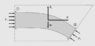# Fluid force at the end of a pipe

• Sat D
From freshman physics, when you do a force balance on object A, you don't include the force object A exerts on object B. You only include the force object B exerts on object A. Didn't they teach you that when they covered Newton's law of action-reaction?In summary, pressure is exerted on the control volume by atmosphere (or the surrounding fluid) and you are trying to find out what is the magnitude and direction of this pressure.f

#### Sat D

Consider the very standard pipe problem used to calculate the force required to hold a pipe with a bend that has some fluid flowing through it, as shown in the image. We use Newton's second law of motion to calculate the external force required. However, why is there pressure acting into the control volume at the right end of the pipe? How do you explain this?
Shouldn't the fluid flowing out generate a pressure (force) acting outward?

#### Attachments

•Capture.JPG
10.8 KB · Views: 399
Remember pressure in a fluid acts in all directions.
Unless the pressure at the outlet is zero, then the pressure there acts outward on the fluid moving into the next section of pipe, tank, or whatever, AND it acts inwards against the fluid behind it. And it also acts sideways on the walls of the pipe in all directions, though it may not be the same on all sides of the pipe if there is a pressure gradient perpendicular to the flow here.

•Chestermiller
Remember pressure in a fluid acts in all directions.
Unless the pressure at the outlet is zero, then the pressure there acts outward on the fluid moving into the next section of pipe, tank, or whatever, AND it acts inwards against the fluid behind it. And it also acts sideways on the walls of the pipe in all directions, though it may not be the same on all sides of the pipe if there is a pressure gradient perpendicular to the flow here.
So why don't we consider the pressure that is pointing outwards while writing out the force balance equations for the pipe?

So why don't we consider the pressure that is pointing outwards while writing out the force balance equations for the pipe?
From freshman physics, when you do a force balance on object A, you don't include the force object A exerts on object B. You only include the force object B exerts on object A. Didn't they teach you that when they covered Newton's law of action-reaction?

In this you considered a control volume and you are trying to calculate what are the forces that are acting on the fluid in the control volume. On the right side the pressure is exerted on the control volume by atmosphere (or the surrounding fluid)